[BACK]Computer Modeling in Engineering & SciencesDOI: 10.32604/cmes.2021.016403

ARTICLE

DEM Simulations of Resistance of Particle to Intruders during Quasistatic Penetrations

State Key Laboratory of Structural Analysis for Industrial Equipment, Dalian University of Technology, Dalian, 116023, China
*Corresponding Author: Shunying Ji. Email: jisy@dlut.edu.cn
Received: 03 March 2021; Accepted: 07 April 2021

Abstract: Based on the discrete element method and hydrostatics theory, an improved Archimedes principle is proposed to study the rules pertaining to resistance changes during the penetration process of an intruder into the particulate materials. The results illustrate the fact that the lateral contribution to the resistance is very small, while the tangential force of the lateral resistance originates from friction effects. Conversely, the resistance of particulate materials on the intruder mainly occurs at the bottom part of the intruding object. Correspondingly, the factors that determine the resistance of the bottom part of the intruding object and the rules pertaining to resistance changes are analyzed. It is found that when the volume density and friction coefficient of the particles and the radius of the bottom surface of the cylindrical intruder are varied, the resistance–depth curve consists of a nonlinear segment and a linear region. The intersection of the two stages occurs at the same location h/R~=0.15±0.055. The slope of the linear stage is determined by the friction coefficient of the particles. Accordingly, the relationship between the slope and the friction coefficient is quantified. Finally, it is shown that the slope is independent of the geometry of the intruder.

Keywords: Particulate materials; improved Archimedes principle; quasistatic resistance; discrete element method

1  Introduction

Granular media exist in natural environments and are extensively used in industrial productions. These types of media include natural particles like grain, and artificial particles like glass beads . Despite extensive studies conducted during the last decade , neither the statics nor the dynamics of particulate materials are sufficiently understood . One of the typical problems is the quasistatic penetration problem that plays a significant role in the static properties of particulate materials  and in the perturbation responses, such as the formation of shear bands [7,8], settlement effects , and the jamming transition . Hence, this study aims to achieve an in-depth understanding of the resistance of particulate materials to intruders during the impact process, and to build a bridge between the static resistance and dynamic fluidity of particles.

To study the resistance of particulate materials to intruders, that reflects the complex physical properties of particulate materials in the process at which intruders impact dry particulate beds [13,14], it is necessary to build an interaction model involving the intruder and the particulate materials. In current research efforts, limited attention is being paid to quasistatic penetration processes [5,15]. Penetration dynamics depends on the intruder’s velocity and the microphysical characteristics of the impact system, such as the particle type, initial filling fraction, and the existence of interstitial fluid. Therefore, scholars a) considered the static or quasistatic effects, based on which the initial intruder velocity was found to be much lower than the sound velocity, and b) described the “slow” dynamics based on the macro force law when the intruder impacted the dry particles [16,17]. Scholars have established an empirical law to describe this process [18,19]: the resistance of the particulate materials with respect to the intruding object is mainly composed of the static resistance Fz, which is related to depth, and the inertial resistance Fv, which is related to velocity . The static resistance Fz is a hydrostatic-like force and is mainly related to the frictional plasticity of particles. The inertial resistance Fv is mainly the viscous force between particles, and is generated by the momentum transfer among them [5,21,22]. The aforementioned studies suggest methods for the analysis of the resistance to the penetration of intruding objects in particulate materials in the presence of quasistatic conditions. These methods are effective in the dynamic modeling of slow motion [19,23,24].

In addition, the quasistatic resistance reflects the macroscopic properties of particulate materials, and there is a close relationship between the macroscopic performance of this type of media and the microscopic mechanism . However, uncertainties lead to various difficulties in the study of the microscopic mechanisms. The phenomenological stages of the research focuses on the characterization of the law of motion of particulate materials . The quasistatic problems are studied based on experiments, such as the distribution of the force chain measured based on photoelastic experiments [5,27] or the tracking of particle positions by a high-frequency camera to obtain the dynamic response of particles . However, owing to the limitations of the current test and measurement methods, the accuracy of the results cannot be guaranteed . Different numerical methods and models can be used in numerical simulations [29,30]. Specifically, the discrete element method (DEM) is the main tool used to simulate particulate materials {}. The continuum-based model is suitable for simulating large asteroid impacts that can form large craters on planet surfaces. This study focuses on the influence of objects on particulate materials at the centimeter scale, where the continuum-based model is not applicable. DEM has been acknowledged to be one of the most effective numerical methods for simulations of particle dynamic behavior, and has been applied in a variety of research fields, such as in landslides, sandstorms, and in grain research . Moreover, research studies have shown that the DEM is feasible for the study of particulate materials.

In this study, a numerical model is constructed to evaluate the penetration of an intruding object into particulate materials in the quasistatic state based on the use of the DEM. The variation of the resistance of the particulate materials with respect to the intruder is discussed based on the hydrostatics theory. The influence of the physical properties of particulate materials on the penetration process is recognized. Finally, the dependence of the resistance change on the intruder geometry is also discussed.

2  Discrete Element Method and Setup of Simulation Model

The spherical element and its nonlinear contact model are employed to calculate the interaction between particles to quantify the structural characteristics of particulate materials and their dynamic response during penetration. The contact forces of discrete elements can be divided into the normal contact force Fn and the tangential contact force Fs, which can be represented by a spring–damper–slider model, as shown in Fig. 1a. Fig. 1b is a contact diagram of the discrete elements i and j. The symbols Cn and Cs are the normal and tangential damping coefficients between the particles. Additionally, nij is the vector from the spherical center i to the spherical center j and is defined as the normal vector of the two particles, whereas sij is the tangential vector between particles. The normal overlap between the particles xnp can be expressed as,

xnp=Ri+Rjdij (1)

where dij=|xi–;xj| is the distance between the centers of the elements i and j. Ri and Rj are the radii of the two elements. Additionally, xi and xj are the coordinate vectors of elements i and j in the global coordinate system.Figure 1: Contact model representation used in the discrete element model. (a) Contact model in normal and tangential directions (b) Contact model in the spherical discrete element

The contact force between particles is mainly composed of elastic and viscous force components, and the sliding friction—that is based on Coulomb’s friction—is also considered. The nonlinear Hertz–Mindlin contact model is used to calculate the elastic force between particles. In the normal direction, interparticle forces include the Hertzian elastic force and the nonlinear viscous force [37,38], expressed as,

Fn=Kn(xnp)3/2+32AKn(xnp)1/2Δx˙np (2)

where x˙np represents the relative velocity of the particles, which can be obtained by the velocity vector difference of the particles. A is the viscous parameter of the particles which is related to the mechanical parameters—such as the deformation modulus, viscous coefficient, and Poisson’s ratio—and can be determined by the resilience coefficient of particle collision at a preset velocity. The normal stiffness coefficient between particles is Kn=34ER, where E=E2(1ν2), and R=RiRjRi+Rj, where E and ν are the elastic modulus and Poisson's ratio of particulate materials, respectively. Based on Mindlin theory and the Coulomb’s friction law, and by ignoring the effect of viscous force, the tangential contact force can be expressed as [39,40],

Fs=min(Fs,sign(Fs)μpFn) (3)

where Fs=Ksxn1/2xs, the tangential stiffness coefficients between particles is Ks=8GR, where G=G2(1ν) and G=E2(1+ν). In this case, G is the shear modulus of particulate materials, and μp is the friction coefficient between particles. If the particles contact the rigid boundary, the boundary can be set as a sphere with infinite radius. The contact detection method can refer the [41,42].

In the nonlinear simplified Hertz–Mindlin (no-slip) contact model, the time step is not larger than the maximum time of contact of two particles. The time step is defined as,

Δt=αtmax (4)

where α is the coefficient by experience, α=0.2 when the coordination number is larger than 4, or α=0.4. The maximum time of contact of two particles is defined as,

tmax=πRmin0.163ν+0.8766ρG (5)

where ρ is the density of the particle, Rmin is the minimum radius of the particle.

The numerical simulation is performed using an in-house C++ code based on the theory described previous. A numerical simulation model is set up, as shown in Fig. 2. The cylindrical intruder penetrates the particulate materials at a low but constant velocity. That is to say, velocity-controlled loading is adopted for the intruder. To obtain effective results, the end point of the study is defined as the instant at which the entire cylinder penetrates into the particulate materials. The particles are dense packing in the container with a width w=440 mm, a length l=440 mm and a height h0=200 mm. The numerical simulation uses 220,000 dry spherical particles with diameters in the range of 2.5–4.0 mm. A geometric particle generation algorithm based on the front advancing method is adopted. The specific theory is detailed in .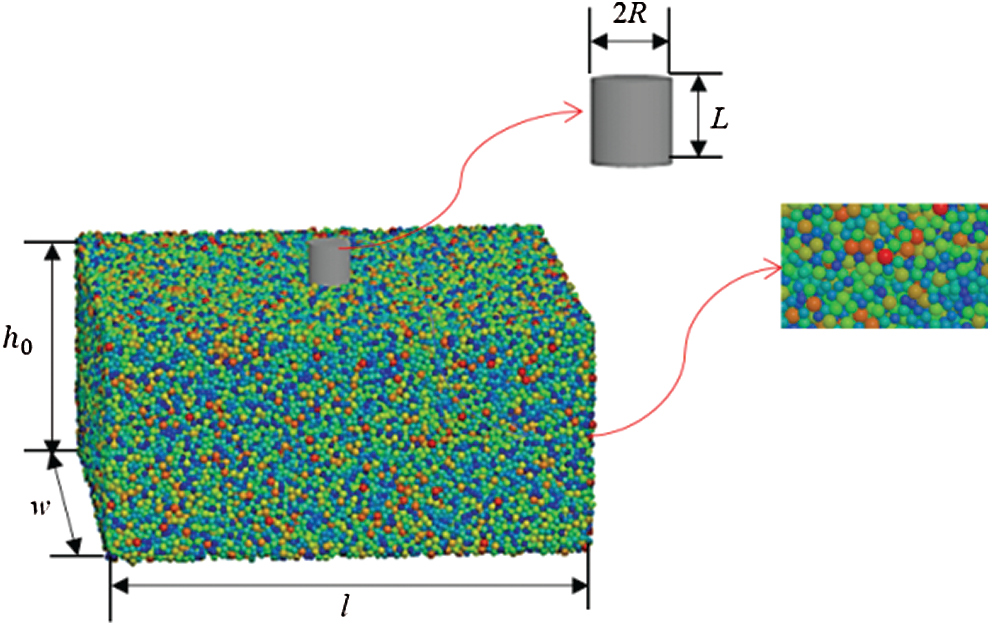Figure 2: Schematic showing the penetration process of the cylindrical intruding object into the particulate materials based on which the numerical simulations were conducted

In the course of the penetration, the existence of the container boundary may generate a strong impact resistance, especially when the cylindrical intruder moves in a direction parallel to the container wall. The boundary effect is mainly caused by the interference inside the stress field of the particulate materials and the side wall [44,45]. To avoid this effect, the size of the container is large enough, and the cylindrical intruder penetration is set to occur in the middle of the container to eliminate the boundary effect . Various particles are simulated by changing the relevant parameters of the particulate materials. The main simulation parameters are listed in Tab. 1.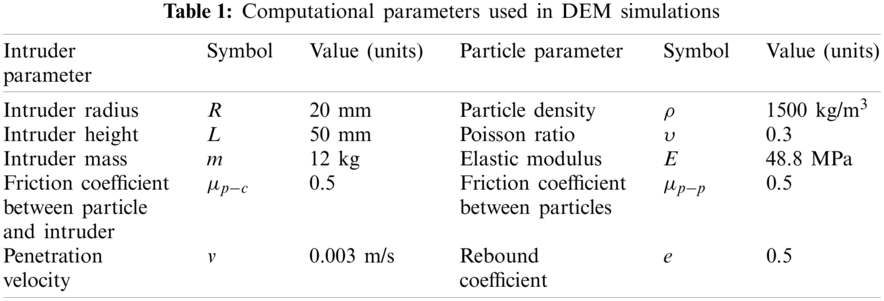3  Analysis of Quasistatic Resistance of Particulate Materials

To ensure that the entire research process is quasistatic, the velocity was chosen to take values in the range of 0.001–0.5 m/s to allow the penetration of the intruder into the particulate materials. Accordingly, the change of Fresultant, that is, the resultant resistance of particulate materials with respect to the intruder for a given penetration velocity is thus obtained, as shown in Fig. 3. It can be observed that the resistance is almost the same at the same depth when the velocity was chosen to take values in the range of 0.001–0.004 m/s. The independence of the velocity with respect to the penetration depth confirms the quasistatic state of the simulation. Therefore, the penetration velocity was chosen to be 0.003 m/s to match the penetration velocity used in the subsequent study.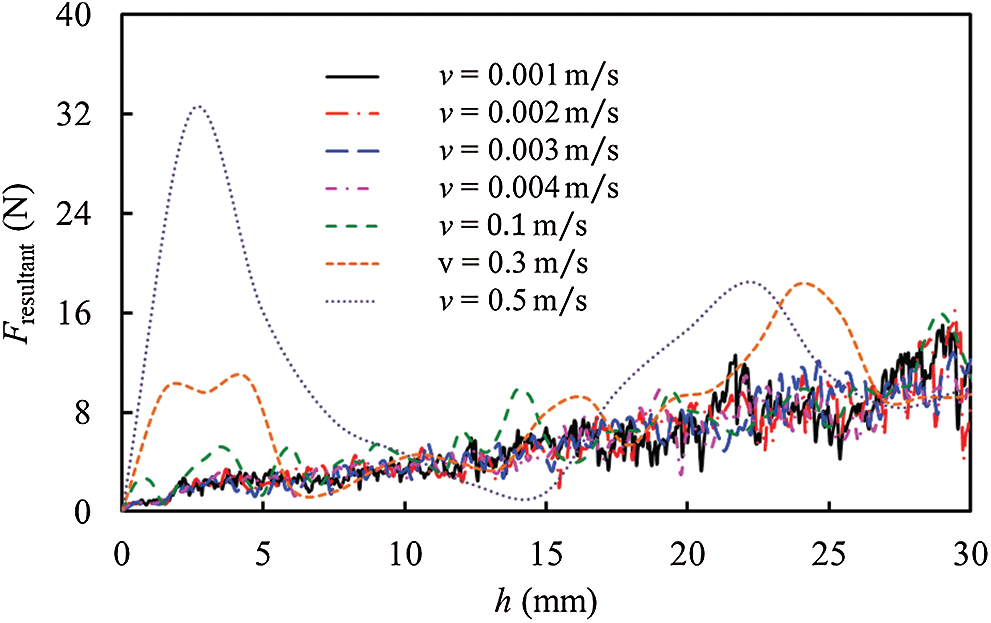Figure 3: Resistance force data associated with the cylindrical intruder at different velocities

During the process of penetration, the particulate materials are mainly in contact with the bottom surface and the sides of the cylindrical intruding object, as shown in Fig. 4. Thus, interactions also occur on the bottom surface and on the sides. Fig. 5 shows the variations of the force Fbottom on the bottom surface and the lateral force Flateral on the cylindrical intruder as a function of the penetration depth. It can be observed that the resistance on the cylindrical intruder is mainly contributed by the bottom surface. The lateral force is small, and as the penetration depth increases, the resistance also increases gradually.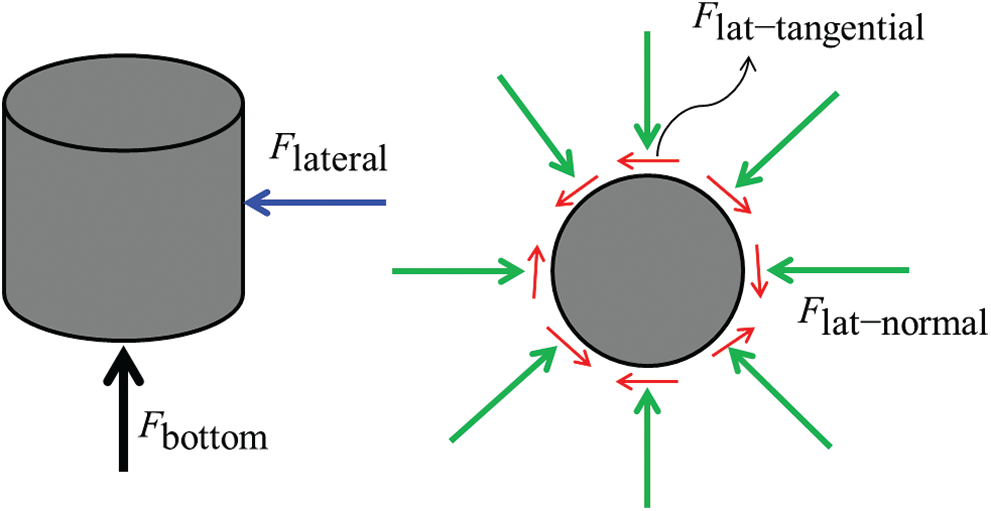Figure 4: Schematic diagram of impact force on the intruding objectFigure 5: Resistance on different surfaces of the intruder as a function of the penetration depth

3.1 Lateral Force Analysis

Even though the lateral force of the intruder is small, it does have an impact on the penetration process. The lateral force can be decomposed into the normal (FLat-normal) and tangential force (FLat-tangential) components, which is depicted in Fig. 4. As shown in Fig. 6, the normal force is obviously greater than the tangential force, and both of them increase as a function of the penetration depth. To study the relationship between them, the results are processed and plotted on logarithmic coordinates, as shown in Fig. 7, and are fitted to curves expressed mathematically according to,

lgFLat-tangential=A1lgh+C1 (6a)

lgFLat-normal=A2lgh+C2 (6b)

In this relation, A1,A2,C1,C2 are coefficients. The above equations can be transformed into,

FLat-tangential=10C1hA1 (7a)

FLat-normal=10C2hA2 (7b)

The values of the coefficients in Eq. (7) obtained by numerical simulations are A1=2.42939, A2=2.43019, C1=4.42298, C2=3.81026. As indicated A1A2. Thus, FLat-tangential/FLat-normal=10C1C2, which means that the ratio of the tangential force to normal force is constant. It is inferred that the tangential force is the friction force generated by the normal force as the tangential force is proportional to the normal force. Therefore, to verify the inference, the relationship between the penetration depth and the tangential force of the lateral resistance was studied at different friction coefficients (as shown in Fig. 8). It is found that when the logarithm of the ratio of resistance to the friction coefficient is used as the coordinate, all the curves almost coincide. This indicates that the tangential resistance denotes the friction attributed to the normal force.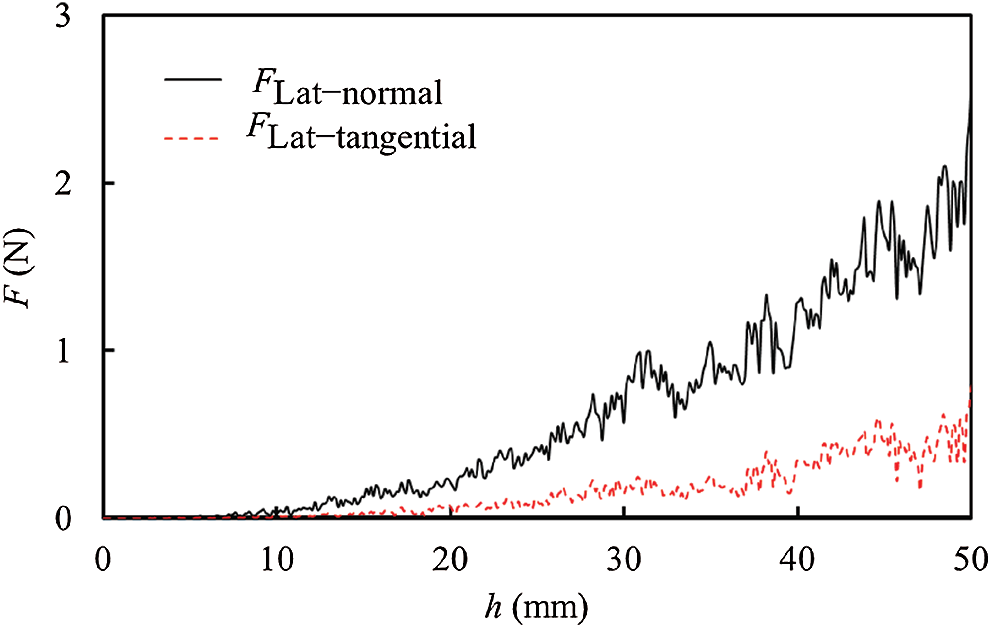Figure 6: Relationships between lateral resistance and penetration depth for the normal and tangential force components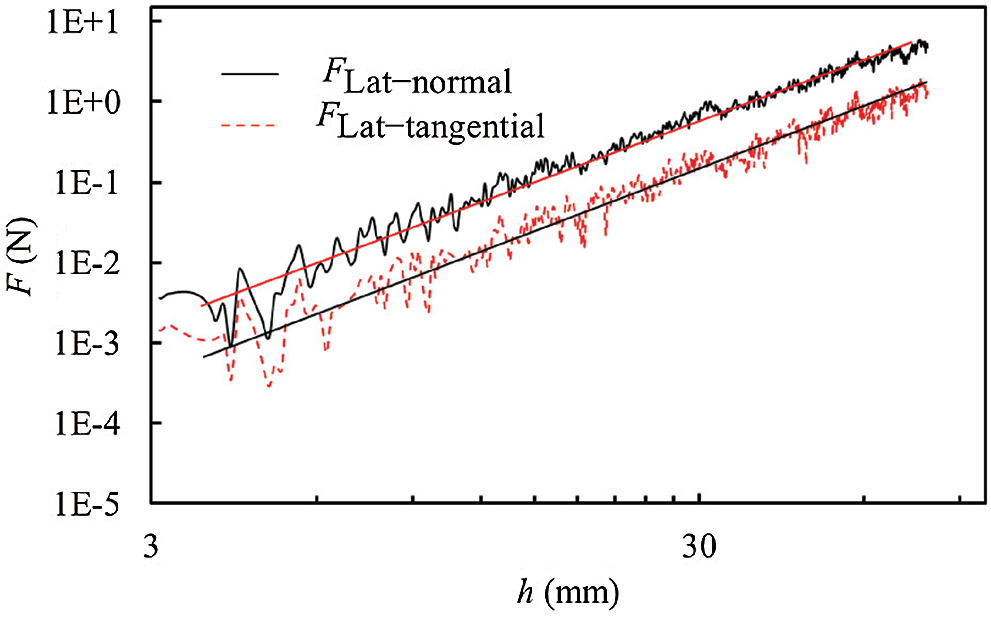Figure 7: Lateral resistance as a function of the penetration depth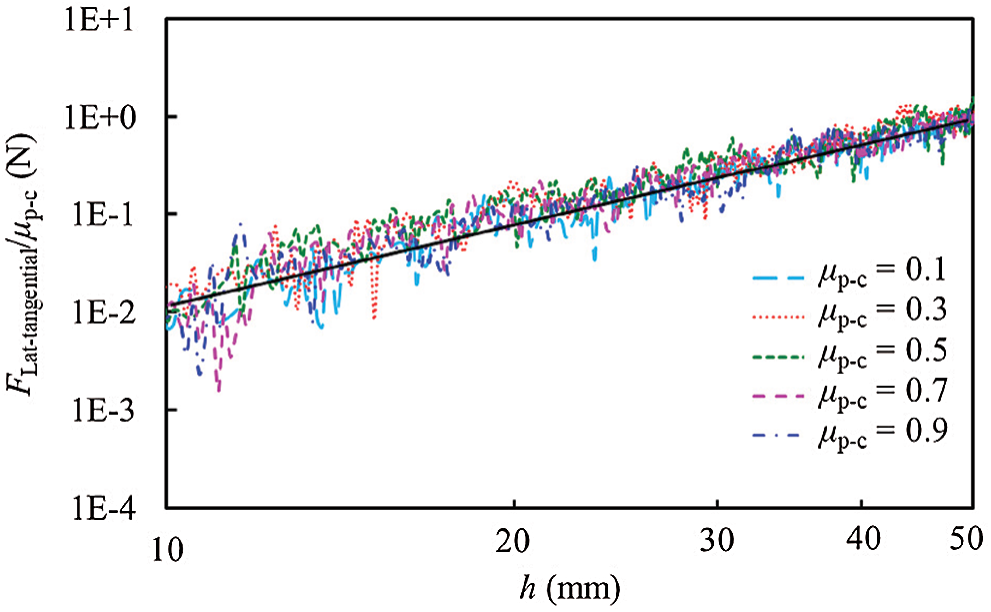Figure 8: Effects of friction coefficients between intruder and particle on the tangential resistances

3.2 Determinants and Shape Dependence of the Force on the Bottom Surface of the Intruder

The bottom surface contributes a lot to the resistance of the cylindrical intruder, as shown in Fig. 5. The resistance–depth curve consists of the initial unstable segment and the linear segment. However, the initial unstable segment is not apparent, so the logarithm of the coordinate is used to obtain a better result. Fig. 9 shows the logarithmic change of the base resistance as a function of depth. The intersection of the two segments is defined as h0. The slope of the linear stage is K. To understand and explain the interaction between particulate materials and cylindrical intruders in the penetration process more clearly, it is necessary to clarify the true meaning of these two intervals and to determine the values of h0 and K.Figure 9: Resistance on the bottom surface (Fbottom) of the cylindrical intruder as a function of the penetration depth

The research conducted herein was based on a quasistatic state. Under quasistatic conditions, nonviscous, dry particulate materials can be regarded as a continuum, with a volume density ρ, and a friction coefficient μpp . Based on the heterogeneity of particulate materials, the resistance on the cylindrical intruders in quasistatic conditions must be related to its penetration volume V. Therefore, simulations were carried out in three groups as follows: cylindrical intruders with different radii were adopted for simulations in the first group, the volume density of the particulate materials was changed in the second group, and the friction coefficient between particulate materials was altered in the third group. The determinants of h0 and the significance of the two intervals were studied based on DEM simulations.

The results are shown in Figs. 1011. When the coordinate is either the logarithm of the ratio of resistance to the area of the bottom surface (S), particle density (ρ), or the radius (R) of the cylindrical intruder, all the curves almost coincide, as shown in Figs. 10b and 11b. Correspondingly, the fluid properties could be characterized owing to the heterogeneity of the particulate materials. In the presence of a quasistatic fluid, the penetration resistance is mainly attributed to the buoyancy of the object.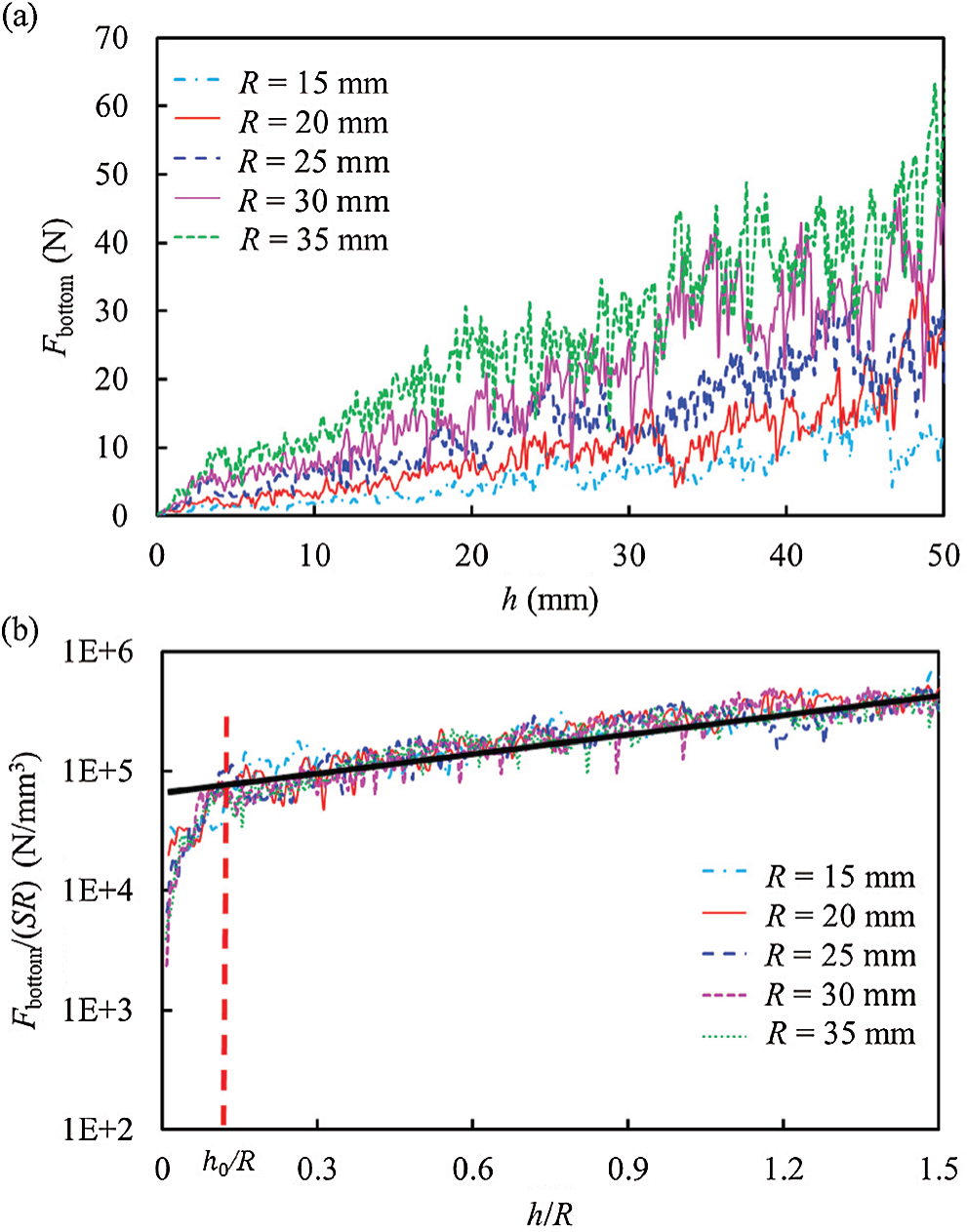Figure 10: Changes in resistance on the bottom surface as a function of the penetration depth for different radii of cylindrical intruders. (a) Fbottom as a function of h (b) Fbottom/(SR) as a function of h/RFigure 11: Plots showing the influences of particle densities. (a) Fbottom as a function of h. (b) Fbottom/ρ as a function of h/R

Therefore, we propose an improved Archimedes principle, whose normalization formula can be written as,

lg(Fbottom/ρgSR)=fun(h/R)if hh0

lg(Fbottom/ρgSR)=f0+Kh/Rif h>h0 (8)

All simulations have yielded the relationship h0/R=0.15±0.055, which is more accurate than the reported value of 0.15±0.06 in . The facts listed above are sufficient to prove the reliability of the calculation.

There is speculation that in the initial stage of the penetration process, the particulate materials under the penetration cylinder formed an additional mass, and subsequently moved with the cylinder. This speculation has been confirmed . The publication reports on findings in quasistatic conditions, and wedge blocks are generated under the circular foundation in which the taper angle of the wedge is 2α=π/2φ, as shown in Fig. 12a. The simulation also shows the wedge blocks, as indicated in Fig. 12b. The velocity vectors of the particulate materials were obtained with numerical simulations. The black dashed area represents the cylindrical impactor. It can be observed that the particles below the cylinder do form a wedge, and their velocities are almost the same as the cylinder’s velocity of 0.003 m/s, as shown in the area enclosed by the red dashed line. This analysis is in good agreement with the observations in [7,47]. Therefore, the unstable segment of the curve corresponds to the transient initial contact between the cylindrical invader and particulate materials, namely the compression process. It is through a linear extension process that the particles under the bottom of the cylinder form a rigid cone. The slope mainly depends on the physical properties of particulate materials. The mass of the rigid cone below the foundation can be expressed as ,

M=πρR33tan(π4φ2) (9)

where φ is the internal friction angle of the particulate materials, which is determined by the particle morphology, dryness, and the friction coefficient between the particles. Based on this theory, it can be determined that the conical angle of the conical body below the cylindrical intruder only depends on the properties of the particulate materials.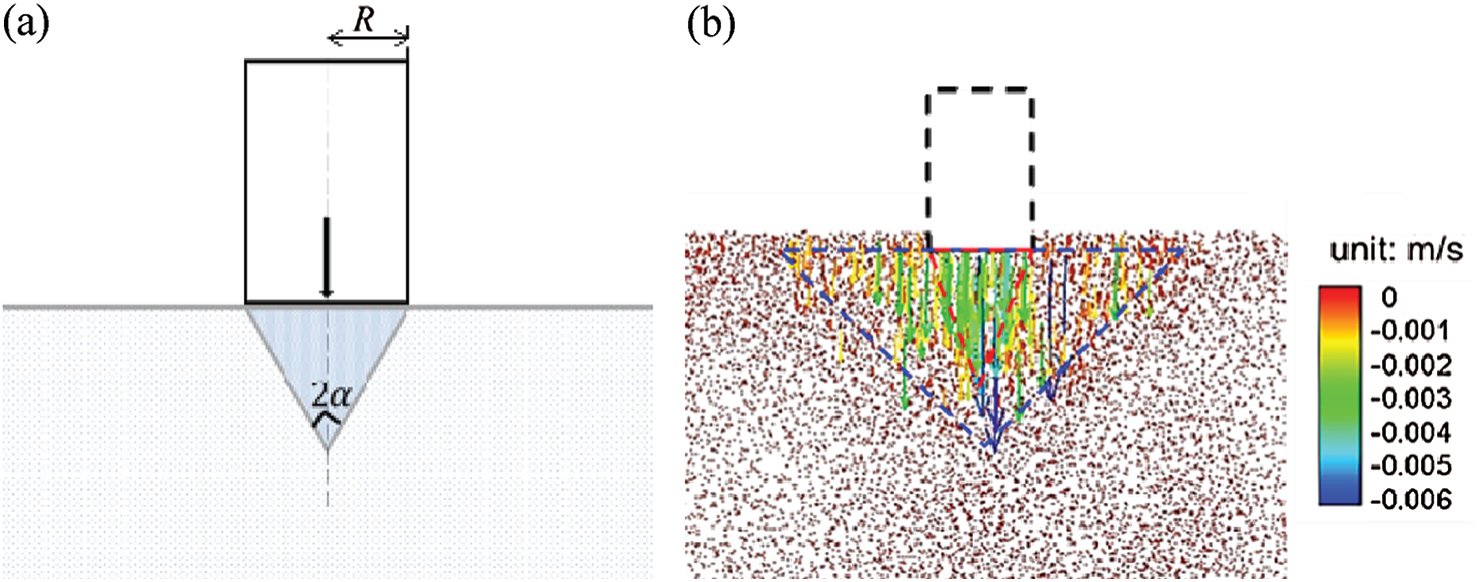Figure 12: Wedge block generated under the circular foundation. (a) Schematic diagram of wedge block (b) Wedge blocks in the simulation result

After the rigid cone is formed, it moves forward in unison with the cylindrical intruder at the same speed. It separates particulate materials and the cylindrical intruder, that is, the resistance on the cylindrical intruder is caused by the contact friction between the particles on the surface of the rigid cone and other particles. It can be concluded that the slope of the linear stage should be related to the friction coefficient between the particulate materials. The slope of the linear stage is defined as K=f(tanφ). This means that the slope of the linear stage K is determined by the angle of the internal friction of the particulate materials . As the particulate materials consist of dry spherical particles, the variable parameter that determines the internal friction angle of particles is only the friction coefficient between the particles. The relationship between resistance and depth at different friction coefficients between particles is shown in Fig. 13a. To determine the linear relationship between the friction coefficient and the slope, different friction coefficients are utilized between the particles to study the dimensionless force–depth curve, as shown in Fig. 13b.

The relationship between the slopes and the friction coefficient is shown in Fig. 14. Accordingly, we can obtain the following relationship as,

K={lg(3.3+41.0μpp)μpp<0.5lg(22.3+3.2μpp)μpp0.5 (10)

This result is significance. It shows that the slope of the linear section is only related to the physical properties of particulate materials. To verify the correctness of the above results, μpp=0.23 is selected for further research. It is found that the slope of the straight line is K=1.139, which is indicated on the fitting curve.

To determine whether h0 and K are dependent on the intruder’s geometrical morphology, prisms with different sections are employed for quasistatic simulations, whereby the equivalent radius is R~=S/π. It is found that compared with the cylindrical intruders, the force variation on the bottom surface with respect to depth is consistent with other intruding object cross-sections, as shown in Fig. 15. This indicates that the shape of the intruder’s cross-section has a minor effect on the penetration process. In addition, in this study, it is not considered that the dimension of the intruder in a certain direction was equal to or smaller than that of the particulate materials, because the mechanical mechanism of such intruder was different from the mechanism of this study.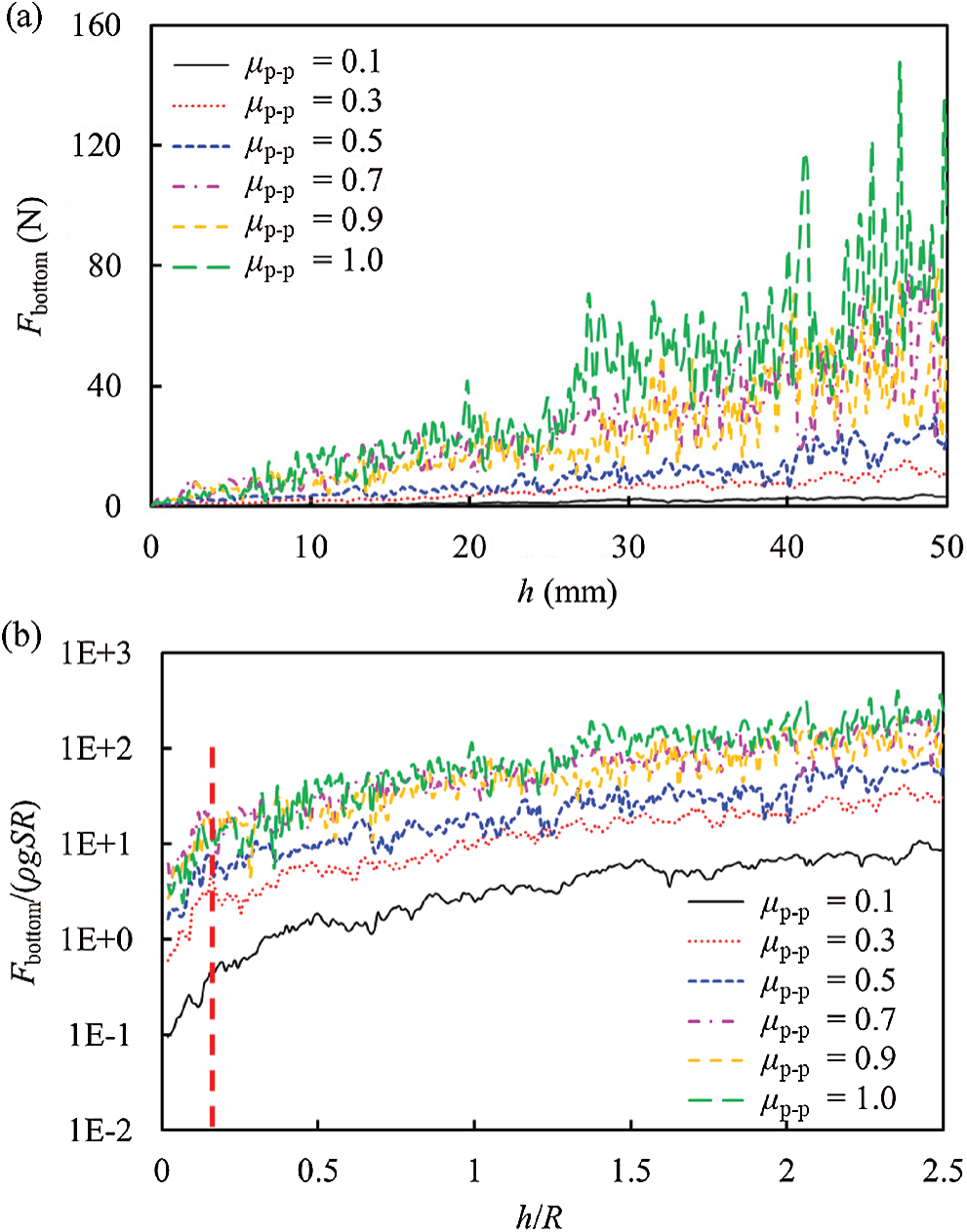Figure 13: Dimensionless force–depth curves at different friction coefficients. (a) Fbottom as a function of h (b) Fbottom/(ρgSR) as a function of h/R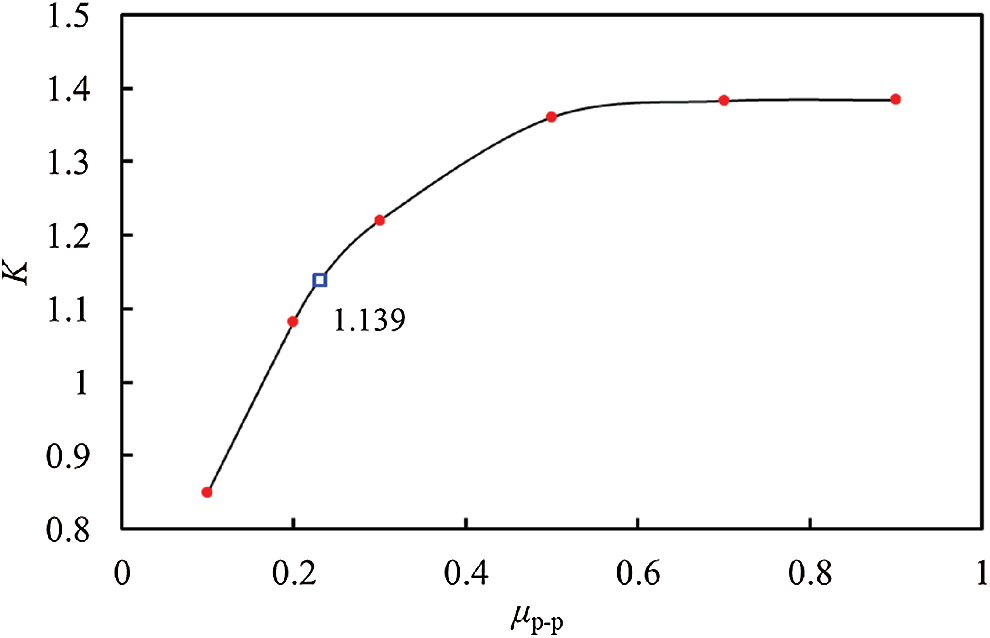Figure 14: Slope variation at the linear stage obtained by numerical calculations as a function of the friction coefficient between the particles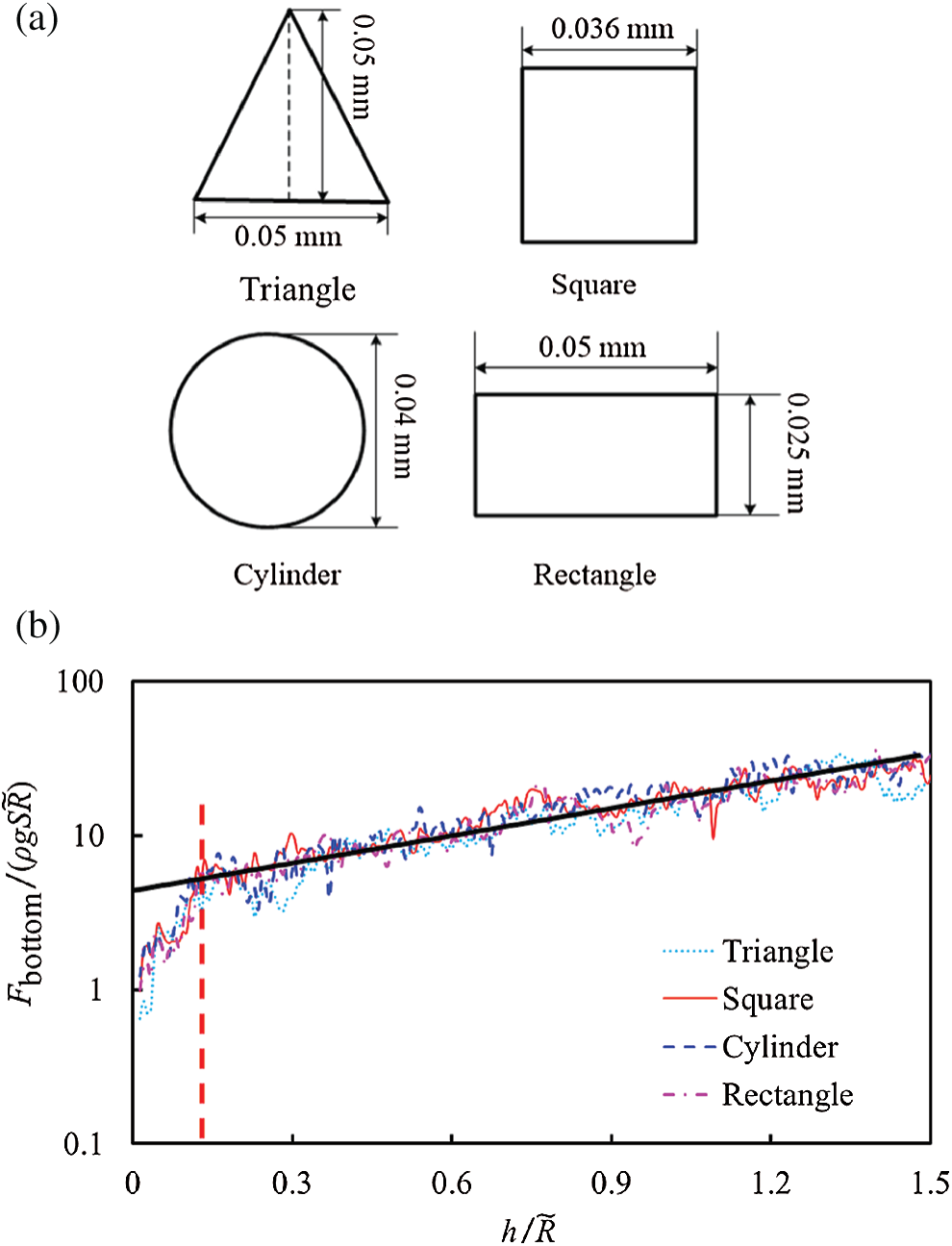Figure 15: Force–depth curve is independent of the intruder’s cross-sectional shape (a) Cross-sectional shapes of different intruding objects (b) Resistances of the bottom surfaces of the intruder as a function of the penetration depth

4  Conclusions

In this study, the penetration resistance of particulate materials following the intrusion of a penetrating object was studied based on DEM simulations and the theory of hydrostatics in quasistatic conditions. During the penetration process, the intruder’s resistance was primarily associated with interactions on its bottom surface. The lateral contribution to the resistance was small. The tangential force of the lateral resistance was the friction effect of the normal component. These results were consistent with the experimental research premise that the influence of the friction between the intruder and the particulate materials could be neglected.

The determinants and the rules pertaining to the changes of resistance on the intruder’s bottom surface were analyzed according to the heterogeneity of particulate materials and based on an improved Archimedes principle. It was found that when the volume density and the friction coefficient of the particles were changed, and the radius of the bottom surface of the cylindrical intruder was altered, the resistance on the cylindrical intruder as a function of the penetration depth was divided into two sections.

Meanwhile, there were two resistance intervals associated with penetration depth changes, and the intersection of the two intervals occurred at h/R~=0.15±0.055. The second interval was based on a linear law, and the slope of the linear stage was not dependent on the geometry of the intruding section, but was mainly determined by the friction coefficient of the particles.

Funding Statement: This study was financially supported by the National Key Research and Development Program of China (Grant No. 2018YFA0605902) and the National Natural Science Foundation of China (Grant Nos. 11572067 and 11772085).

Conflicts of Interest: The authors declare that they have no conflicts of interest to report regarding the present study.

References

1.  1.  Zhuo, C. F., Yao, W. J., Wu, X. S., Feng, F., Xu, P. (2016). Research on the muzzle blast flow with gas-particle mixtures based on eulerian–eulerian approach. Journal of Mechanics, 32(2), 185–195. DOI 10.1017/jmech.2015.44.
2.  2.  Ambroso, M. A., Kamien, R. D., Durian, D. J. (2005). Dynamics of shallow impact cratering. Physical Review E, 72(4), 41305–41308. DOI 10.1103/PhysRevE.72.041305.
3.  3.  Royer, J. R., Corwin, E. I., Eng, P. J., Jaeger, H. M. (2007). Gas-mediated impact dynamics in fine-grained granular materials. Physical Review Letters, 99(3), 38003–38006. DOI 10.1103/PhysRevLett.99.038003.
4.  4.  Jeng, F. S., Wang, T. T., Li, H. H., Huang, T. H. (2011). Influences of microscopic factors on macroscopic strength and stiffness of inter-layered rocks—revealed by a bonded particle model. Journal of Mechanics, 24(4), 379–389. DOI 10.1017/S1727719100002501.
5.  5.  Kang, W., Feng, Y., Liu, C., Blumenfeld, R. (2018). Archimedes’ law explains penetration of solids into granular media. Nature Communication, 9(1), 1101–1109. DOI 10.1038/s41467-018-03344-3.
6.  6.  Sakamura, Y., Komaki, H. (2011). Numerical simulations of shock-induced load transfer processes in granular media using the discrete element method. Shock Waves, 22(1), 57–68. DOI 10.1007/s00193-011-0347-6.
7.  7.  Han, E., Peters, I. R., Jaeger, H. M. (2016). High-speed ultrasound imaging in dense suspensions reveals impact-activated solidification due to dynamic shear jamming. Nature Communication, 7(12243), 12243–12250. DOI 10.1038/ncomms12243.
8.  8.  Namazian, Z., Najafi, A. F., Mousavian, S. M. (2016). Numerical simulation of particle-gas flow through a fixed pipe, using one-way and two-way coupling methods. Journal of Mechanics, 33(2), 205–212. DOI 10.1017/jmech.2016.53.
9.  9.  Huang, L., Ran, X., Blumenfeld, R. (2016). Vertical dynamics of a horizontally oscillating active object in a two-dimensional granular medium. Physical Review E, 94(6), 62906–62912. DOI 10.1103/PhysRevE.94.062906.
10. 10. Vinutha, H. A., Sastry, S. (2016). Disentangling the role of structure and friction in shear jamming. Nature Physics, 12(6), 578–584. DOI 10.1038/nphys3658.
11. 11. Bi, D., Zhang, J., Chakraborty, B., Behringer, R. P. (2011). Jamming by shear. Nature, 480(7377), 355–358. DOI 10.1038/nature10667.
12. 12. Peters, I. R., Majumdar, S., Jaeger, H. M. (2016). Direct observation of dynamic shear jamming in dense suspensions. Nature, 532(7598), 214–221. DOI 10.1038/nature17167.
13. 13. Bester, C. S., Behringer, R. P. (2017). Collisional model of energy dissipation in three-dimensional granular impact. Physical Review E, 95(3), 32906. DOI 10.1103/PhysRevE.95.032906.
14. 14. Lin, J. H., Chang, K. C. (2015). Particle dispersion simulation in turbulent flow due to particle–particle and particle-wall collisions. Journal of Mechanics, 32(2), 237–244. DOI 10.1017/jmech.2015.63.
15. 15. Brzinski, T. A., Mayor, P., Durian, D. J. (2013). Depth-dependent resistance of granular media to vertical penetration. Physical Review Letters, 111(16), 168002–168008. DOI 10.1103/PhysRevLett.111.168002.
16. 16. Katsuragi, H., Durian, D. J. (2007). Unified force law for granular impact cratering. Nature Physics, 3(6), 420–423. DOI 10.1038/nphys583.
17. 17. Li, Y., Ji, W. (2013). Acceleration of coupled granular flow and fluid flow simulations in pebble bed energy systems. Nuclear Engineering and Design, 258, 275–283. DOI 10.1016/j.nucengdes.2013.02.032.
18. 18. Askari, H., Kamrin, K. (2016). Intrusion rheology in grains and other flowable materials. Nature Materials, 15(12), 1274–1279. DOI 10.1038/nmat4727.
19. 19. Aguilar, J., Goldman, D. I. (2016). Robophysical study of jumping dynamics on granular media. Nature Physics, 15(12), 278–284. DOI 10.1038/nphys3568.
20. 20. Clark, A. H., Behringer, R. P. (2013). Granular impact model as an energy-depth relation. Europhysics Letters, 101(6), 64001–64007. DOI 10.1209/0295-5075/101/64001.
21. 21. Garry, J. R. C., Towneti, M. C., Ba, A. J., Zarnecki, J. C., Marcou, G. (1999). The effect of ambient pressure and impactor geometry on low speed penetration of unconsolidated materials. Advances in Space Research, 23(7), 1229–1234. DOI 10.1016/S0273-1177(99)00189-1.
22. 22. Luding, S. (2008). Cohesive, frictional powders: Contact models for tension. Granular Matter, 10(4), 235–246. DOI 10.1007/s10035-008-0099-x.
23. 23. Uehara, J. S., Ambroso, M. A., Ojha, R. P., Durian, D. J. (2003). Low-speed impact craters in loose granular media. Physical Review Letters, 90(19), 194301–194305. DOI 10.1103/PhysRevLett.90.194301.
24. 24. Li, C., Zhang, T., Goldman, D. I. (2013). A terradynamics of legged locomotion on granular media. Science, 339(6126), 1408–1440. DOI 10.1126/science.1229163.
25. 25. Ambroso, M. A., Santore, C. R., Abate, A. R., Durian, D. J. (2004). Penetration depth for shallow impact cratering. Physical Review E, 71(5), 51305–51311. DOI 10.1103/PhysRevE.71.051305.
26. 26. Ma, L., Huang, D., Chen, W., Jiao, T., Sun, M. et al. (2017). Oscillating collision of the granular chain on static wall. Physics Letters A, 381(5), 542–548. DOI 10.1016/j.physleta.2016.12.011.
27. 27. Peters, J. F., Muthuswamy, M., Wibowo, J., Tordesillas, A. (2005). Characterization of force chains in granular material. Physical Review E, 72(4), 41307. DOI 10.1103/PhysRevE.72.041307.
28. 28. Wang, D., Zheng, X. (2013). Experimental study of morphology scaling of a projectile obliquely impacting into loose granular media. Granular Matter, 15(6), 725–734. DOI 10.1007/s10035-013-0446-4.
29. 29. Goldman, D. I., Umbanhowar, P. (2008). Scaling and dynamics of sphere and disk impact into granular media. Physical Review E, 77(2), 21308–21311. DOI 10.1103/PhysRevE.77.021308.
30. 30. Gravish, N., Goldman, D. I. (2010). Force and flow transition in plowed garticle media. Physical Review Letters, 105(12), 128301–128304. DOI 10.1103/PhysRevLett.105.128301.
31. 31. Kuang, S. B., Lamarche, C. Q., Curtis, J. S., Yu, A. B. (2013). Discrete particle simulation of jet-induced cratering of a granular bed. Powder Technology, 239, 319–336. DOI 10.1016/j.powtec.2013.02.017.
32. 32. Wada, K., Senshu, H., Matsui, T. (2006). Numerical simulation of impact cratering on granular material. Icarus, 180(2), 528–545. DOI 10.1016/j.icarus.2005.10.002.
33. 33. Ciamarra, M. P., Lara, A. H., Lee, A. T., Goldman, D. I., Vishik, I. et al. (2004). Dynamics of drag and force distributions for projectile impact in a granular medium. Physical Review Letters, 92(19), 194301. DOI 10.1103/PhysRevLett.92.194301.
34. 34. Li, Y., Dove, A., Curtis, J. S., Colwell, J. E. (2016). 3D DEM simulations and experiments exploring low-velocity projectile impacts into a granular bed. Powder Technology, 288, 303–314. DOI 10.1016/j.powtec.2015.11.022.
35. 35. Cleary, P. W. (2001). Recent advances in DEM modelling of tumbling mills. Minerals Engineering, 14(10), 1295–1319. DOI 10.1016/S0892-6875(01)00145-5.
36. 36. Weng, M. C., Cheng, C. C., Chiou, J. S. (2013). Exploring the evolution of lateral earth pressure using the distinct element method. Journal of Mechanics, 30(1), 77–86. DOI 10.1017/jmech.2013.73.
37. 37. Wang, C., Deng, A., Taheri, A., Zhao, H. H., Li, J. (2019). Modelling particle kinetic behaviour considering asperity contact: Formulation and DEM simulations. Granular Matter, 21(2), 16–31. DOI 10.1007/s10035-019-0868-8.
38. 38. Su, Y., Cui, Y., Ng, C. W. W., Choi, C. E., Kwan, J. S. H. (2019). Effects of particle size and cushioning thickness on the performance of rock-filled gabions used in protection against boulder impact. Canadian Geotechnical Journal, 56(2), 198–207. DOI 10.1139/cgj-2017-0370.
39. 39. Bahaaddini, M., Sharrock, G., Hebblewhite, B. K. (2013). Numerical direct shear tests to model the shear behaviour of rock joints. Computers and Geotechnics, 51(4), 101–115. DOI 10.1016/j.compgeo.2013.02.003.
40. 40. Ji, S., Shen, H. H. (2006). Characteristics of temporalspatial parameters in quasisolid-fluid phase transition of granular materials. Chinese Science Bulletin, 51(6), 646–654. DOI 10.1007/s11434-006-0646-y.
41. 41. Han, K., Feng, Y. T., Owen, D. R. J. (2007). Performance comparisons of tree-based and cell-based contact detection algorithms. Engineering Computations, 24(2), 165–181. DOI 10.1108/02644400710729554.
42. 42. Di Renzo, A., Di Maio, F. P. (2004). Comparison of contact-force models for the simulation of collisions in DEM-based granular flow codes. Chemical Engineering Science, 59(3), 525–541. DOI 10.1016/j.ces.2003.09.037.
43. 43. Li, Y., Ji, S. (2018). A geometric algorithm based on the advancing front approach for sequential sphere packing. Granular Matter, 20(4), 58–69. DOI 10.1007/s10035-018-0833-y.
44. 44. Stone, M. B., Bernstein, D. P., Barry, R. (2004). Stress propagation getting to the bottom of a particle medium. Nature, 427(6972), 503–505. DOI 10.1038/427503a.
45. 45. Seguin, A., Bertho, Y., Gondret, P. (2008). Influence of confinement on granular penetration by impact. Physical Review E, 78(1), 10301–10304. DOI 10.1103/PhysRevE.78.010301.
46. 46. Nelson, E. L., Katsuragi, H., Mayor, P., Durian, D. J. (2008). Projectile interactions in granular impact cratering. Physical Review Letters, 101(6), 68001–68006. DOI 10.1103/PhysRevLett.101.068001.
47. 47. Viswanathan, K., Mahato, A., Murthy, T. G., Koziara, T., Chandrasekar, S. (2015). Kinematic flow patterns in slow deformation of a dense granular material. Granular Matter, 17(5), 553–565. DOI 10.1007/s10035-015-0576-y.
48. 48. Wu, X., Zhong, S., Ling, D., Chen, Y. (2012). Model test study of vertical impact of space lander footpad. Rock and Soil Mechanics, 33(4), 1045–1050. DOI 10.16285/j.rsm.2012.04.007.This work is licensed under a Creative Commons Attribution 4.0 International License, which permits unrestricted use, distribution, and reproduction in any medium, provided the original work is properly cited.